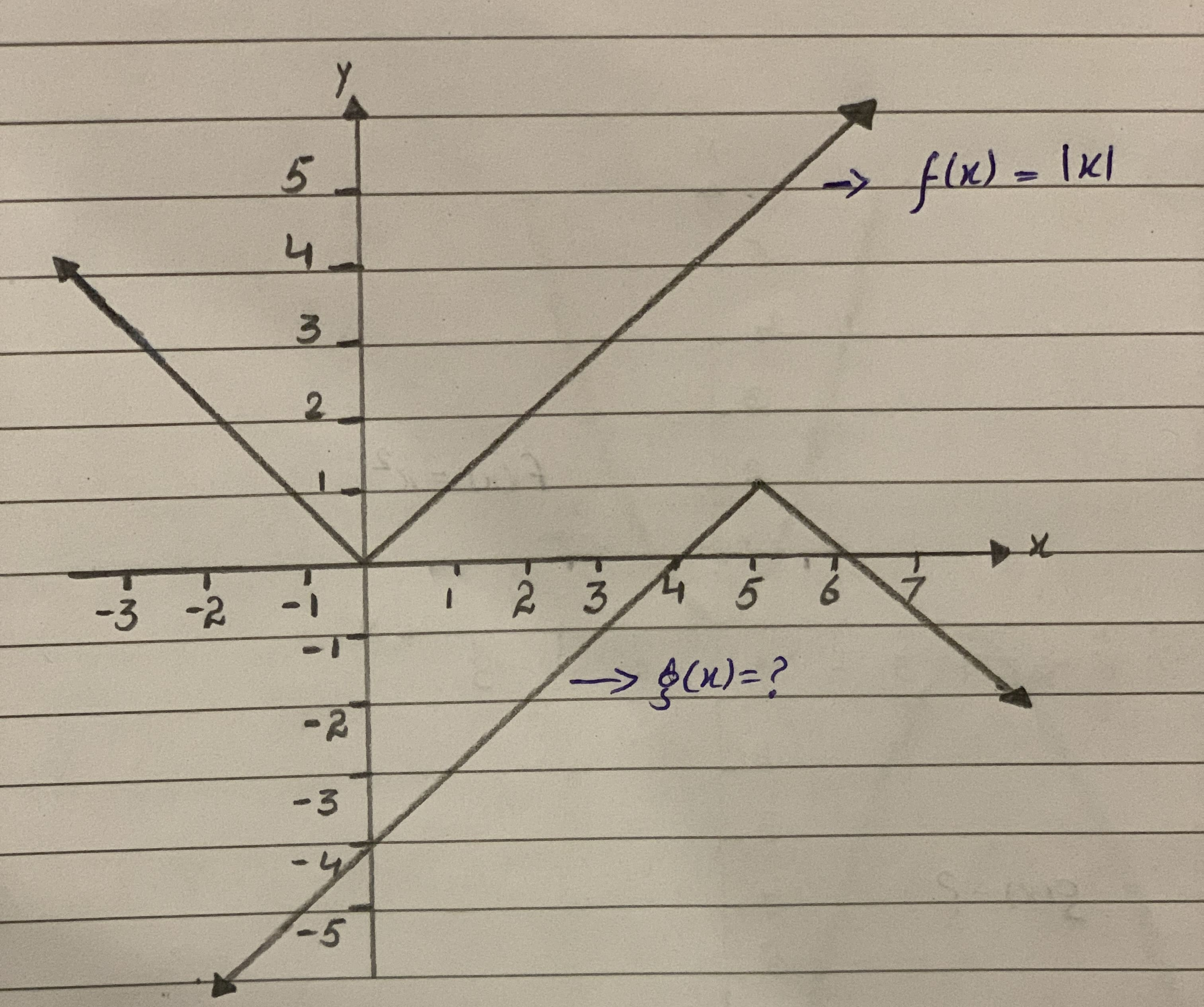# Functions f and g are graphed in the same rectangular coordinate system (see attached herewith). If g is obtained from f through a sequence of transformations, find an equation for g<img src="13310200082.JPG">ringearV 2021-02-27 Answered

Functions f and g are graphed in the same rectangular coordinate system (see attached herewith). If g is obtained from f through a sequence of transformations, find an equation for g• Questions are typically answered in as fast as 30 minutes

### Plainmath recommends

• Get a detailed answer even on the hardest topics.
• Ask an expert for a step-by-step guidance to learn to do it yourself.l1koVWe know function g(x) is obtained from f(x)
transformations we use for g(x) are
$$f(x)g=|x|$$ reflection of f(x) with x-axis
$$\Rightarrow -∣x∣$$
then we shift our resultant graph by 5 units towards left on x axis
$$\Rightarrow -∣x−5∣$$
then at last shift the resultant graph by 1 unit towards upward
$$g(x)=-x∣x−5∣∣+1$$